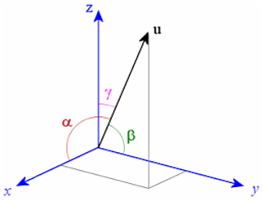#### A line makes equal angles with co-ordinate axes. Direction cosine of this line are Option 1)Option 2)Option 3)Option 4)As learnt in

Direction Cosines -

Ifare the angles which a vector makes with positive X-axis,Y-axis and Z-axis respectively thenare known as diresction cosines, generally denoted by (l,m,n).- whereinwhere,Option 1)This option is incorrect

Option 2)This option is correct

Option 3)This option is incorrect

Option 4)This option is incorrect